# Electronics and Communication Engineering - Automatic Control Systems

Exercise : Automatic Control Systems - Section 3
1.
Lag compensation increases bandwidth of the system.
True
False
Explanation:

Lag compensation improves steady state behaviour.

2.

Assertion (A): The time response of the system with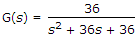will not have any overshoot for unit step input.

Reason (R): A critically damped system does not have any overshoot to a unit step input.

Both A and R are correct and R is correct explanation of A
Both A and R are correct but R is not correct explanation of A
A is correct but R is wrong
R is correct but A is wrong
Explanation:

System in A is overdamped.

3.
The polar plot of the given figure is for the term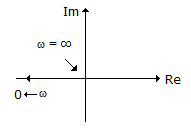(jω)2
1 + (jω)2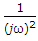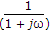Explanation:

For the term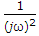, magnitude is 0 at ω = ∞ and infinite at ω = 0. Moreover phase angle is -180°.

4.
For the unity feed back system of the given figure, the closed loop transfer function is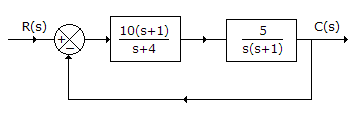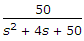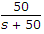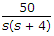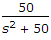Explanation: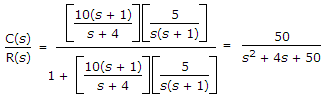.

5.
The effect of negative feedback on distortion and bandwidth because
both are decreased
both are increased
distortion is reduced and bandwidth is increased
distortion is increased and bandwidth is decreased